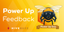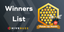# Parametric Calculus: Areas: Example 2: Cow Grazing Area: Method 1

in Threespeak2 months ago (edited)

In this video I go over a very interesting example, which is the somewhat famous “cow (or goat or another farmyard animal) grazing area” problem which involves determining the area in which the cow can graze if it is tied to a circular silo. There are several ways of solving this area problem, and in this video I look at Method 1, which involves using the parametric equations of the involute curve, and which were obtained from my last video on Parametric Calculus: Example 1: Involute; so make sure to watch that video! This initial method is actually VERY tedious, so much so that I had to use an online integral calculator in the middle of this video because otherwise this video would be about an hour long. I may do another video going over the integral manually, which involves using integration by parts multiple times, so stay tuned for that video!

The total grazing area is the area between the involute curve and the silo, as well as the area of a large half-circle to the left of the silo. The area between the involute and the silo is obtained by the basic area method of infinite rectangles which sum up as an integral, but an interesting result occurs when calculating the area. Because the involute is not a one-to-one function, we need to break the involute area into two parts, but then these two parts actually sum to exactly the same result if we instead just solved the integral for the whole range of the function, regardless of it being not a one-to-one function! Thus the integral automatically subtracts the excess area as I showed in this video! I may investigate this further to see if I can come up with a more general formula so stay tuned for that too!

While this method of solving the cow grazing area is very tedious, the final result is nonetheless very compact and is 5(π3)(r2)/6. I will go over a much simpler way of solving this area in a later video, which involves summing up infinite areas of sectors of circle, like in polar coordinates, and thus we don’t need to use parametric equations. Understanding the different ways of solving the same problem is what makes the overall field of mathematics truly remarkable. Thus make sure to watch this video and the ones that follow on how to solve this “grazing area” problem!

View video notes on the Hive blockchain: https://peakd.com/mathematics/@mes/video-notes-parametric-calculus-areas-example-2-cow-grazing-area

Related Videos:

Parametric Calculus: Example 1: Involute of a Circle: https://youtu.be/NIGw_dlEzQ4
Parametric Calculus: Surface Area Part 1: https://youtu.be/4bMEIf6WD8M
Parametric Calculus: Arc Length Part 1: https://youtu.be/AWvJDK-m6wQ
Parametric Calculus: Areas: https://youtu.be/XdplYV61xlM
Parametric Calculus: Tangents: https://youtu.be/deQwD2o0Sas
Parametric Equations and Curves: https://youtu.be/Kd3XF4LZoFE
Parametric Calculus: Surface Area: Example 1: Sphere: https://youtu.be/S0o4feoSp80
Trigonometric Identity: sin(2x) = 2sin(x)cos(x): http://youtu.be/IaZFDmVtyyU .

SUBSCRIBE via EMAIL: https://mes.fm/subscribe

DONATE! ʕ •ᴥ•ʔ https://mes.fm/donate

Like, Subscribe, Favorite, and Comment Below!

MES Truth: https://mes.fm/truth
Official Website: https://MES.fm
Hive: https://peakd.com/@mes

Email me: [email protected]

Free Calculators: https://mes.fm/calculators

BMI Calculator: https://bmicalculator.mes.fm
Mortgage Calculator: https://mortgagecalculator.mes.fm
Percentage Calculator: https://percentagecalculator.mes.fm

Free Online Tools: https://mes.fm/tools

iPhone and Android Apps: https://mes.fm/mobile-apps

▶️ 3Speak

Sort:

Hi. Just informing You and Your readers that from now, one can invest in CCFound crypto startup without KYC (up to 1000 Euro); earlier KYC was obligatory so it is good change.

Next selling round starts in 10 days.

Interesting. Thanks for the heads up! May throw in some cash lol

Congratulations @mes! You have completed the following achievement on the Hive blockchain and have been rewarded with new badge(s) :You received more than 9000 HP as payout for your posts and comments.Your next payout target is 10000 HP.The unit is Hive Power equivalent because your rewards can be split into HP and HBD

If you no longer want to receive notifications, reply to this comment with the word `STOP`Feedback from the October 1st Hive Power Up DayHive Power Up Month Challenge - Winners List